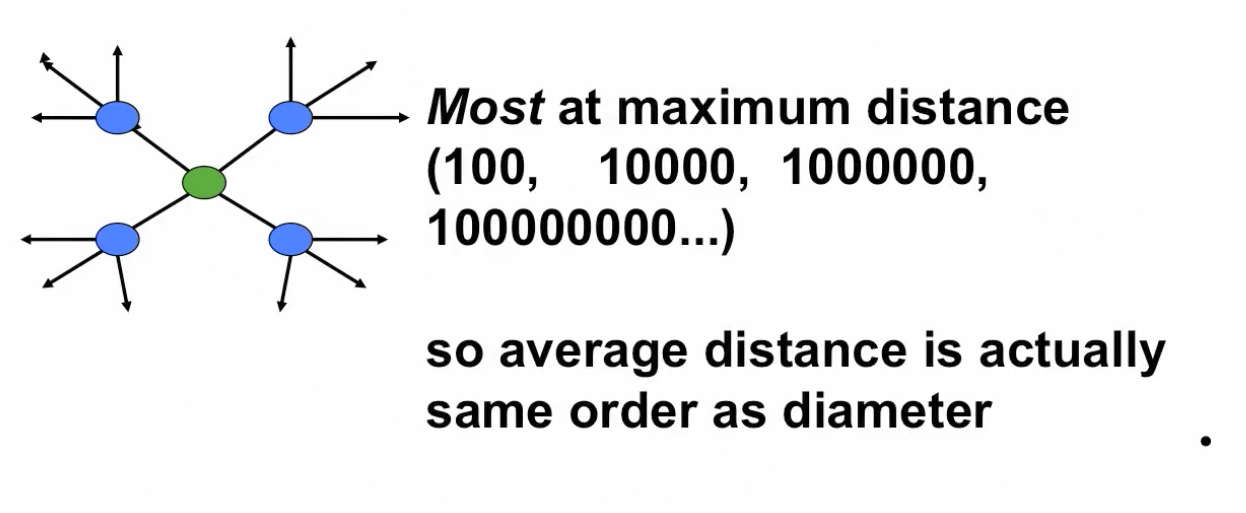# Theorem on Network Structure¶

If $d(n) \ge (1+\epsilon)log(n)$ some $\epsilon \ge 0$ and $\frac{d(n)}{n} \rightarrow 0$:

then for large n, average path length and diameter of this G(n, p) graph are approximately proportional to $\frac{log(n)}{log(d)}$

$$\frac{AvgDist(n)}{log(n) / log(d(n))} \rightarrow ^p 1$$

same for diameter

• Chernoff Bounds:

• X is binomial variable then $$Pr(E[X]/3 \leq X \leq 3E[X]) \ge 1 - e^{-E[X]}$$

https://en.wikipedia.org/wiki/Chernoff_bound

"X" can be viewed as number of links end up with

the important thing here is that if we're looking at a binomial random variable, so if you are just flipping coins with some probability p and then counting how many come up- so how many come up it as a given- so imagine I'm looking at a particular node and it's forming all of its links and we ask how many links does it end up with, well, the probability that the number of links ends up with is between three times the expected number, and a third of the expected number is at least one minus e raised to the minus expected number. So if I'm expected to have a hundred friends, then the chance that I'll have somewhere between 100 over three and 300 is one minus e to the minus 100. Well, this is getting very close to one, right? So it's as if you expect me to have 100 friends, then very close to one is the probability that I'm going to be- have at least 33 and smaller than 300. So Chernoff bounds begin to say, "Okay, there's a very high probability that you're going to be within a factor of three of what the expectation is.

• Chernoff Bounds: Links binomial limplies Probability that node has degree close to average: $$Pr(d/3 \leq d_i \leq 3d) \ge 1 - e^{-d}$$

$$Pr(d/3 \leq all.degrees \leq 3d) \ge 1 - e^{-d}$$

(missing steps: degrees not quite ind.)

• If $d \ge (1+\epsilon)log(n)$ then $$Pr(d/3 \leq all.degrees \leq 3d) \ge (1 - 1/n^{1+\epsilon})^n$$

$\rightarrow exp(-n^{-\epsilon}) \rightarrow 1$

• So: $$Pr(d/3 \leq all.degrees \leq 3d) \rightarrow 1$$

• If $d \ge (1+\epsilon)log(n)$ then with $prob \rightarrow 1$: $$\frac{log(n)}{log(3d)} \leq l \leq \frac{log(n)}{log(d/3)}$$ $l$ is the distance

Avg distance and diameter:

• Large d: log(3d) & log(d/3) tend to log(d)

• $$\frac{log(n)}{log(3d)} \leq l \leq \frac{log(n)}{log(d/3)}$$

• $$\frac{log(n)}{log(d)} \approx l$$

• some links may double back

• most nodes until the last step are still not reached, so most links still reaching new nodes!
• After k steps reached around $d^k$ nodes and $n-d^k$ still unreached
• if $k \leq log(n)/log(d)$ then $n - d^k$ (much) bigger than $d^k$, so most nodes that link to are still unreached...